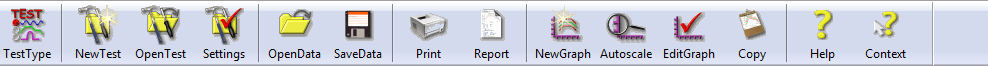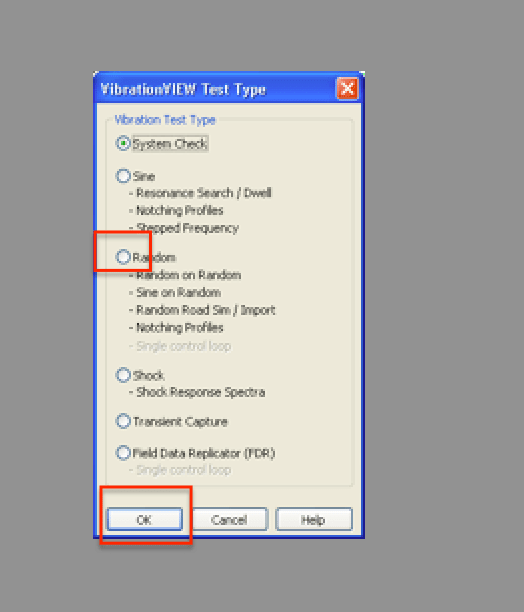# VN0004 - Quick Tricks for Entering a Random Test

## Abstract

VibrationVIEW will automatically calculate the third unknown value in a given Random profile when two of the following values are known: G2/Hz for Frequency 1, Slope, G2/Hz for Frequency 2.

### Problem

A customer called in with the following random specification and was not sure how to enter the test into VibrationVIEW:

• Line 1: .052 G2/Hz from 80Hz to 500Hz
• Line 2: ‐6db/octave from 20Hz to 80Hz
• Line 3: ‐6db/octave from 500Hz to 2000Hz
• Line 4: Total 7.4 G RMS

### Solution

A random test specification can come in many formats. Vibration Research has taken the best of the formats and combined them for easy test entry in VibrationVIEW.

Step 1
Select “New Test” from the VibrationVIEW toolbarStep 2Step 3
Insert additional lines into the “Table” tab until the number of lines and the number of given frequencies in the test specification match. In this case there are four frequencies so we would insert 4 lines.
Step 4
Enter the frequencies from the test specification in ascending order in the frequency column. 20, 80, 500, and 2000 Hz.Step 5
Now we can begin entering the known G2/Hz values:
.052 G2/Hz at 80Hz and
.052 G2/Hz at 500Hz
Step 6
The click‐order is important here to automatically calculate the unknown value
Look at the screen shot on the right and notice the triangle formed by the Amplitude and Slope entry boxes. Always start in the known amplitude box. In this case we would click in row 2 (80Hz) column 2 (.052 G2/Hz) Then click in the second known box of the three boxes formed by the triangle. In this example we also know the slope to be ‐6 from 20‐ 80 Hz so we would click from the known amplitude box to the slope box and enter ‐6. You will see that the third box of the triangle – the unknown G2/Hz value – turns yellow. Once we click out of the slope box after entering ‐6 the yellow box is automatically calculated. This would also work if given two G2/Hz values and an unknown slope. You would simply enter in the first known amplitude and then the second. The third box in the triangle will always be auto‐calculated based on the two known values.Step 7
You can verify the test was entered correctly by comparing the 1G RMS calculated by VibrationVIEW and the given overall G RMS from the test specification. There will almost always be some rounding error when comparing the values. You can see that the test spec gives 7.4 G RMS and VibrationVIEW calculates 7.402. This is within an expected range given any rounding variations and we can assume that the test has been entered as required.